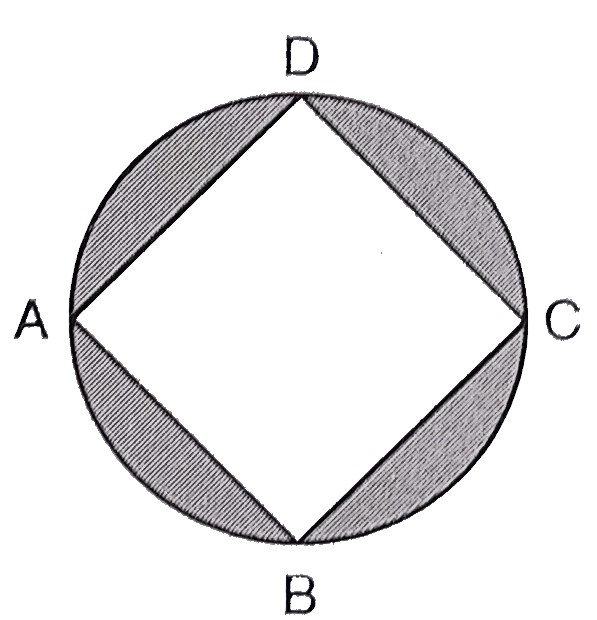"
">

# In the figure below, $A B C D$ is a square with side $2 \sqrt{2} \mathrm{~cm}$ and inscribed in a circle. Find the area of the shaded region. (Use $\pi=3.14)$"

Given:

$A B C D$ is a square with side $2 \sqrt{2} \mathrm{~cm}$ and inscribed in a circle.

To do:

We have to find the area of the shaded region.

Solution:

From the figure,
$ABCD$ is a square.

$AD = 2\sqrt2\ cm$

Join $AC$,

This implies,

Diagonal of the square $\mathrm{AC}=\sqrt{2} \times \mathrm{AD}$

$=\sqrt{2} \times 2\sqrt{2} \mathrm{~cm}$

$=2 \times 2$

$=4 \mathrm{~cm}$

Diagonal of the square $=$ Diameter of the circle

This implies,

Radius of the circle $=\frac{4}{2}$

$=2 \mathrm{~cm}$

Therefore,

Area of the shaded region $=$ Area of circle $-$ Area of square

$=\pi r^{2}-(\mathrm{AD})^{2}$

$=3.14 \times(2)^{2}-(2\sqrt{2})^{2}$

$=3.14 \times 4-4\times2$

$=4(3.14-2)$

$=4 \times 1.14$

$=4.56 \mathrm{~cm}^{2}$

The area of the shaded region is $4.56\ cm^2$.

Updated on: 10-Oct-2022

696 Views